# Reverse Image Lookup Out Of The Box

What is a reverse image search?

It is where you give me an image and I find a bunch of images that look like the one that you gave me. So, I thought that I would give that a quick try. But I am out of time to train one from scratch. So I decided to cheat. I used transfer learning. Now the cool thing about transfer learning is that I am not going to train the model at all. Usually, you fine tune the model to your domain. But I don’t have time for that.

So I just used an image classification algorithm out of the box. I didn’t train it at all, I just used the default values for the parameters. The surprising thing is that it worked really well. However, I still sucked on Kaggle’s leader board.

### Let the cheating begin

After I got the data into google colab, I needed a function that would give me an embedding for the images that I would feed to it. Luckily, keras comes with a bunch of models ready to go for transfer learning, they have VGG, Resnet, and a bunch of others. Usually the way that you deal with these models is to take the embedding and attach that to a small neural net that you train to classify the images. Today we take the embeddings as they are, and try to find the nearest neighbors in the embedding space.

```from keras.applications.resnet50 import ResNet50
from keras.preprocessing import image
from keras.applications.resnet50 import preprocess_input
import numpy as np
from urllib.request import urlopen
from PIL import Image

model = ResNet50(weights='imagenet', include_top=False)

def get_features(url):
try:
img_file = urlopen(url)
im = Image.open(img_file)
except:
output = *(256*256*3)
output = np.array(output).reshape(256,256,3).astype('uint8')
im = Image.fromarray(output).convert('RGB')

im2 = im.resize((224, 224), Image.ANTIALIAS)
x = image.img_to_array(im2)
x = np.expand_dims(x, axis=0)
x = preprocess_input(x)

features = model.predict(x).reshape(1,2048)
return features
```

In the embedding space, that I get for free, just from loading the model, if two images are close together, they should look similar, and theoretically, should have similar classes. So we’re just going to grab the embeddings for a bunch of images, let’s call it 10,000 to start off with. And then we’ll look at the k-nearest neighbors for that image. In our case the top 10-ish. We’ll actually look at 2 through 10, in case it tries to grab itself.

Okay so let’s take a look at what that actually looks like in terms of code. The first thing that we need to do is to convert a bunch of images to data using the function above, so we’ll write another function that ultimately is just a wrapper around the previous function. This new function is going to look at 10,000 images at a time and convert them to a handy pandas dataframe.

```import pandas as pd
pd.DataFrame().to_csv('drive/resnet50_features.csv')
def convert_data(j):
df = pd.read_csv('drive/resnet50_features.csv')
X = []
i = 0
for url in urls[10000*j:10000*j+10000]:
if i == 1:
df = df.drop('Unnamed: 0',axis=1)
v=str(round(100*(i)/10000,4))
print('\r','Status: ',v,'% Complete for Group ',str(j),end='')
X.append(get_features(url))
i += 1
df = pd.DataFrame(np.array(X).reshape(i,2048))
df.to_csv('drive/resnet50_features{0}.csv'.format(j),index=None)

return df
```

And for speed of implementation, we’ll just look at the first 10,000 images.

```df = convert_data(0)
```

;

Now, we just need a method for finding similar images. So what we’re going to do is just use plain old k-nearest neighbors to find the top 10-ish closest images to the one we’re querying on. First things first, let’s train the KNN algorithm using the features that we extracted from resnet.

```from sklearn.externals import joblib
from sklearn.neighbors import KDTree
kdt = KDTree(df, leaf_size=30, metric='euclidean')
joblib.dump(kdt, 'drive/kdtree.pkl')
```

With the model trained, we are ready to try to find similar images to a query image. The following function takes one of our images in the form of a url and displays that image. Then it finds the top 9 closest matches that it can find and displays them in a 3X3 grid of images. I then use simple voting by the most similar images to predict classes in the competition. It didn’t do as well as I would hope with just 10,000 images in the dataset, so I’m increasing that number now. Anyway, here is the function.

```import matplotlib.pyplot as plt
import matplotlib.image as mpimg
def display_matches(url):
x = get_features(url)
try:
img_file = urlopen(url)
im = Image.open(img_file)
except:
output = *(256*256*3)
output = np.array(output).reshape(256,256,3).astype('uint8')
im = Image.fromarray(output)
im2 = im.resize((224, 224), Image.ANTIALIAS)
x2 = np.asarray(im2)
plt.imshow(x2)
plt.grid(False)
plt.show()
neighbors = kdt.query(x, k=10, return_distance=False)
print(neighbors[1:])
matches=[]
for neighbor in neighbors[1:]:
try:
img_file = urlopen(urls[neighbor])
im = Image.open(img_file)
except:
output = *(224*224*3)
output = np.array(output).reshape(224,224,3).astype('uint8')
im = Image.fromarray(output)
im2 = im.resize((224, 224), Image.ANTIALIAS)
temp = np.asarray(im2)
matches.append(temp)

matches=np.array(matches).reshape(3,3,224,224,3).transpose(0,2,1,3,4).reshape(3*224,3*224,3)
plt.imshow(matches)
plt.grid(False)
plt.show()
return(None)
```

All in all even at just 10,000 training images, with default weights for the resnet, the model seems to do a good job. For example here is one image that I queried: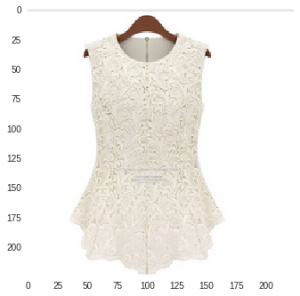I got this back as my top 9 predicted matches.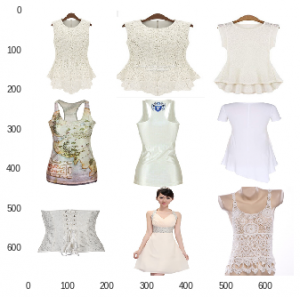That looks awesome! My top 3 matches even have wood hangers! That isn’t so bad! Okay, let’s take a look at another one.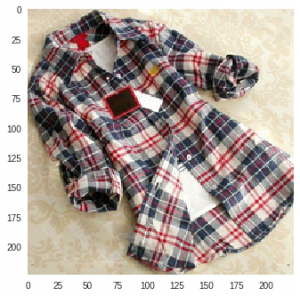And what the algorithm gave back as my top 9 matches: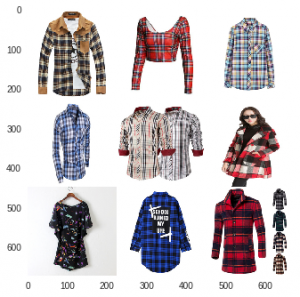Not bad I got a bunch of flannel back, but I have a mixture of Men’s and Women’s  clothes which will probably screw up my predictions. Also I found a couple of examples that were just terrible looking at random query results. For example when I queried for this: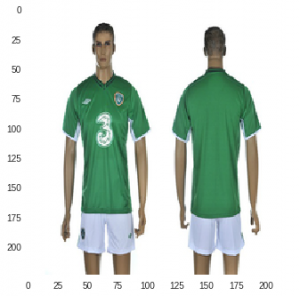I would have expected to see some soccer jersey, or just sports apparel, but what I got was this.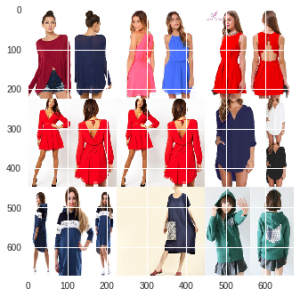Women wearing dresses?! I really need more data to feed into this thing. Fortunately, this is the exception rather than the rule. Generally, it seems to be doing a good job, but it can obviously improve.

Published